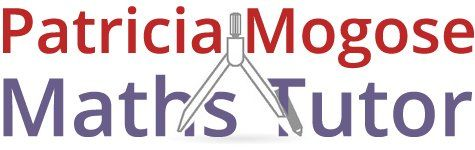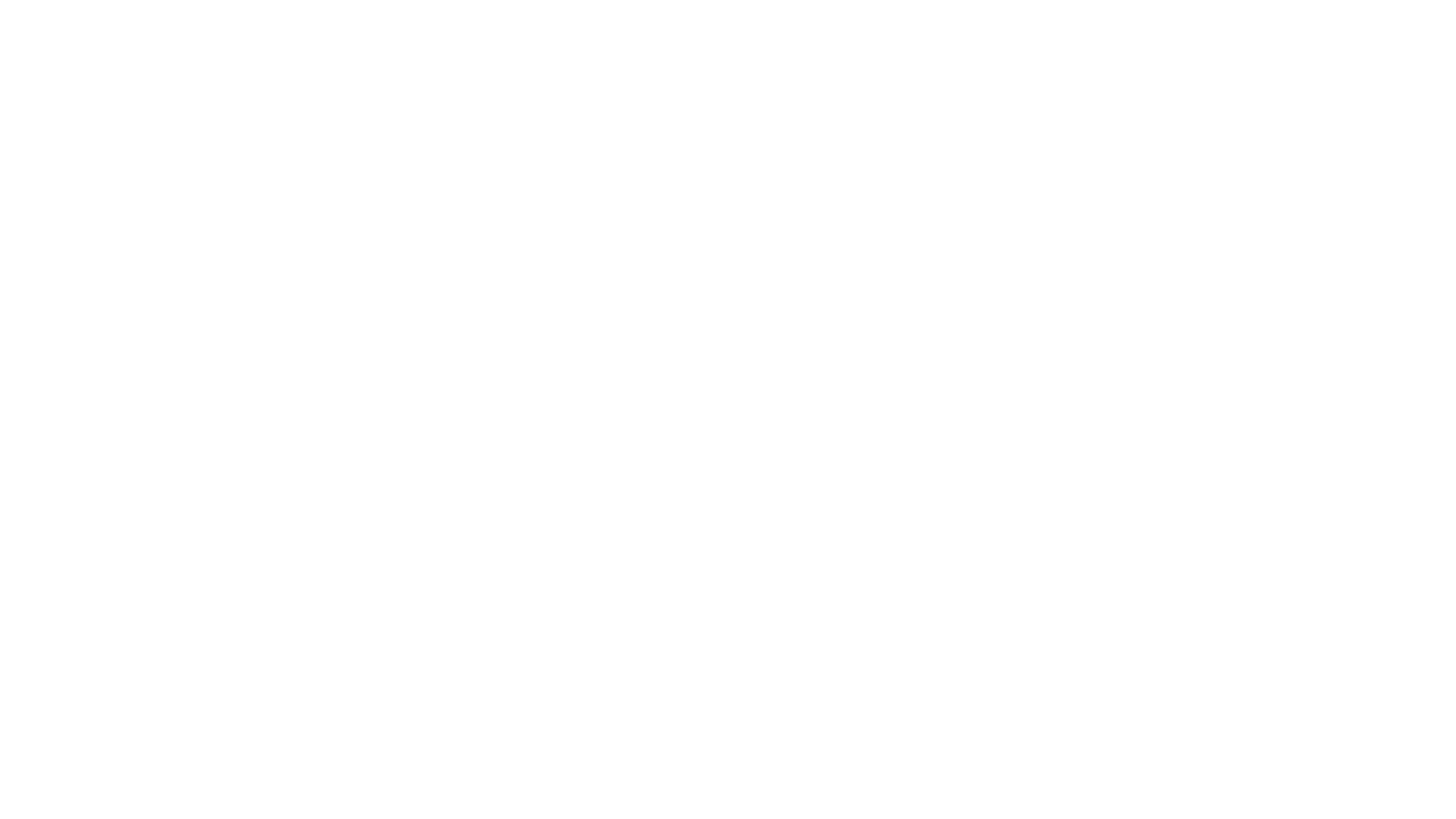For maths tuition with Patricia, call:
01733 265 440
patricia.mogose@sky.com

# Effective private tuition servicesin Peterborough

Make a difference in your child’s life with private tuition services. At Patricia Mogose Maths Tutor in Peterborough, I am committed to offering a quality learning experience. My tuition classes can be tailored to your child’s specific needs. I also offer primary, secondary and A-level maths tuition. Get in touch with me today.

#### BSc Honours and PGCE qualified### Year 7 classes

• Understanding BIDMAS (order of the operations)
• All operations using 2 and more decimal places, plus manipulation of numbers in 4 or more digits
• Multiplying and dividing by varying powers of 10
• Finding equivalent fractions
• Converting fractions, decimals and percentages between each other
• Calculator work with brackets, squares and square roots
• Rounding numbers to whole numbers, nearest 10, 100, 1000 etc. also 1 and 2 decimal places
• LCM and HCF
• Prime numbers and triangular numbers
• Angles on a straight line and at a point, angle calculation in a triangle
• Areas of compound shapes, perimeter and volume
• Averages and ranges, including use of frequency tables
• Ratios and proportions
• Using letters to represent numbers, starting algebra
• simple algebraic expressions
• Understanding inverse operations
• Number sequences from word rules
• Plotting straight line graphs### Year 8 maths tuition

• Division by decimal
• Larger powers and roots
• Percentage increase and decrease
• Simplification of ratios, sometimes containing units of measurement
• Ratio sharing into 3 or more parts
• Manipulation of negative/positive numbers with all 4 operations
• Expressing a number as a product of prime factors, also to find theL.C.M. and H.C.F.
• Simplifying algebraic expressions by collection of like terms
• Solving linear equations
• Finding the nth term of a sequence
• Graphs of real-life situations
• Function mapping
• Angles in parallel lines, opposite angles at a vertex
• Volumes of prisms, surface areas of cuboids
• Areas of parallelogram and trapezium
• 4 quadrant co-ordinates in graphs
• Further transformations: translation and enlargement### Classes for year 9

• Significant figure and decimal place rounding
• Calculator use for more complex arithmetical calculation, using brackets, powers, roots and fractions
• Using ratios to find 1:n or n:1, and the idea of direct proportion
• Speed, distance and time relationships
• Solving inequalities and indicate solution on number line
• Expansion of brackets and simplification
• Multiplying and dividing indices
• Solving equations by ‘trial and improvement’
• Rearranging equations(changing the subject)
• Solving simultaneous equations by algebraic and graphical means
• nth term of quadratic sequences
• Equations of straight line graphs
• Plotting graphs of quadratic functions
• Reciprocals
• Circles, circumference and area
• Maps and scale drawing
• Bearings
• Pythagoras theorem
• Trigonometry
• Tessellations
• Interior and exterior angles of polygons
• Congruency and similarity in shapes
• Means, modes and medians in grouped frequency data